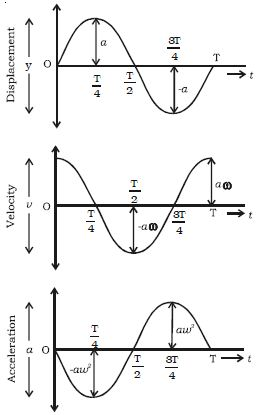Physics

# Graphical representation of Simple Harmonic Motion

Graphical representation of Simple Harmonic Motion:

Graphical representation of displacement, velocity and acceleration of a particle vibrating simple harmonically with respect to time t is shown in Figure.(i) Displacement graph is a sine curve. The maximum displacement of the particle is y = ± a

(ii) The velocity of the vibrating particle is maximum at the mean position i.e u = ± a ω and it is zero at the extreme position.

(iii) The acceleration of the vibrating particle is zero at the mean position and maximum at the extreme position (i.e) ± a ω2.

The velocity is ahead of displacement by a phase angle of π/2. The acceleration is ahead of the velocity by a phase angle π/2 or by a phase π ahead of displacement. (i.e) when the displacement has its greatest positive value, acceleration has its negative maximum value or vice versa.